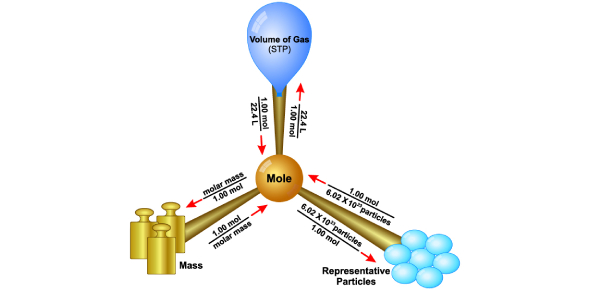# Moles And Molar Mass Test Questions And Answers

10 Questions | Attempts: 2347
ShareSettingsWelcome to our moles and molar mass test questions and answers quiz, where you'll be asked to calculate the number of moles and molar masses to the given atoms or molecules. Basically, the molar mass of a compound represents the mass of one mole of that substance. If your concepts are clear and can easily solve the numerical, then no one can stop you from securing a good score on this quiz. Shall we begin it then? All the best, buddy!

• 1.
Find molar mass of AlCl3
• A.

97g/mol

• B.

97g

• C.

133.5g/mol

• D.

133.5g

• 2.
Calculate the molar mass of CaCOH.
• A.

69.09 g/mol

• B.

68.09 g/mol

• C.

69.10 g/mol

• D.

68.09 g/mol

• 3.
Which of the following would have more atoms?
• A.

1 mole of Au

• B.

1 mole of Si

• C.

1 mole of Li

• D.

None, all are equal

• 4.
What is the mass in grams of 0.172 moles of NaHCO3?
• A.

14.5 grams

• B.

14.4 grams

• C.

18 grams

• D.

None of the above

• 5.
How many moles of hydrogen are in H2?
• A.

0.01 moles

• B.

0.1 moles

• C.

1 moles

• D.

2 moles

• 6.
Calculate the molar mass of Cr4(P2O7)3.
• A.

728.8 g/mol

• B.

729.9 g/mol

• C.

729.8 g/mol

• D.

None of the above

• 7.
Calculate the number of moles of H2 in 10 gram of H2.
• A.

3 moles

• B.

5 moles

• C.

0.5 moles

• D.

5.5 moles

• 8.
Find the amount of mole in 0.1g of hydrogen gas (H2)
• A.

0.1 mol

• B.

0.1g

• C.

0.05 mol

• D.

0.05g

• 9.
Find the number of particles in 0.01 moles of sodium?
• A.

0.01 x 6.02 x 1023 particles

• B.

0.02 x 6.02 x 1023 particles

• C.

23 x 6.02 x 1023 particles

• D.

0.01 particles

• 10.
Find the mass of 0.01mol of hydrogen gas (H2)
• A.

0.01 g/mol

• B.

0.01g

• C.

0.02 g/mol

• D.

0.02g

## Related TopicsBack to top
×

Wait!
Here's an interesting quiz for you.